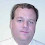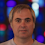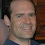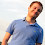## Monday, July 30, 2012

### Six Questions about unnatrual and natural mathematical objects

Today (Monday) I pose some questions. In my next post (Tuesday) I will post the answers
that I know (some I do not). Some questions are a matter of opinion
in terms of what you consider natural.

1. Is there a subset of [0,1] that is uncountable and has measure 0?
2. Is there such a set that is natural?
3. Is there a function that is continuous everywhere and differential nowhere?
4. Is there such a function that is natural?
5. Is there a function that has derivative 0 at almost every points of [0,1] even though it is strictly increasing?
6. Is there a natural such function?

I request that all comments be NON-anonymous.  (I'll tell you why when I post the answers I know on Tuesday.)

1.Do you want spoilers?
1. Yes.
2. Yes.
3. Yes.
4. I was going to say no, but then I decided yes.
5. On this one, I'm guessing that the answer must be no.
6. If 5 is actually yes, then this is absolutely no.

1.Some spoilers are fine and some are not- which is why I
want people to post non-anonymously so if I block someone
I can email them why I blocked them.

All will be clear tommorow.

2.I'm not gonna touch the "natural" questions with a 10 foot pole because matter of opinion of what you consider natural in these problems is an understatement.

#3 and #5 are stereotypical trig trivia Qs.

#3 is the definition of ye olde wiserstraus eqn (sorry almost surely misspelled his name) the point of which was an infinite summation of a bunch of cosine terms. Gut level believing it is pretty easy, proving is somewhat harder. Generally speaking doing calculus on infinite summations is often entertaining.

#5 is ye old integral of trig functions trivia Q where you want a sine wave tilted so it never decreases and has a ridiculously high frequency so it hits zero deriv rather often in 0,1. One big problem with this design is "zero at almost every point" is arguably much different than has infinitely many zeros and unfortunately having infinitely MORE non-zeros than zeros in between the zeros. Many zeros vs high ratio of zeros vs non-zeros, so to speak. Also demanding [0,1] makes it harder to use a reciprocal in the trig function (to get infinitely many 0 derivs) than (0,1]. So its one of those superficially easy Qs that rapidly gets harder.

I would assume question #1 is also a trivia question but I do not recall seeing it before. At least not at this time of morning.

Trivia questions as in if you've seen the wiserstraus before you'll know the answer instantly, if not, well, then probably not. Also trivia in that I have no idea what to do with a wiserstraus other than confuse or hopefully interest people.

3.1. Yes.
2. Yes.
3. Yes. I remembered covering this in my analysis class.
4. No. The answer I had in mind for 3 doesn't work for 4.
5. Yes. Round down to the nearest member of the Cantor set.
6. #6 seems natural to me.

4.1-6: Yes (The Cantor set is quite natural. The Wiener process is the most natural stochastic process :-) )

5.I'd say that the answer to #6 is yes. Just let r_n be a natural enumeration of the fractions in the interval (0,1). Define f(x) to be the sum of {1/n^2: x > r_n}. This isn't continuous, but it is strictly increasing, and seems natural to me. The proof that it has derivative zero almost everywhere is a fairly tasty exercise, and I'll leave it at that for now. It's not that hard to make a continuous example from this, but doing so obscures the differentiability argument.

6.1. Yes.
2. No.
3. Yes.
4. No.
5. Yes.
6. No

I'm familiar with the pathological examples for 1,3,5 (I had in mind: Cantor, Weierstrass, and the Devil's staircase) - but I can't say either is very "natural" to me.

7.1. yes
2. no
3-6 yes.

My definition: If I can write a stochastic process that is nice and useful which has the property almost surely, then I have to go with it being natural.

8.If we take "natural" to mean "is a simple intuitive model of a phenomenon observed in nature", then I agree with Konstantin that brownian motion for 3. is as natural as anything.

Or we can take natural to mean "the first construction that comes to mind works". Or at least that the construction does not feel like magic after one has seen it. I have a problem with evaluating that: once you have seen a technique it stops being magic (with the exception of some techniques I guess). For example, using brownian motion (as opposed to weierstrass) as an example of 3. to me is an infinite version of the natural argument, "if you want to construct a generic object, take a random one". In some sense being differentiable is highly non-generic property, and one expects to avoid it by somehow carefully sampling a random continuous function. But I cannot comment on the cantor set and devil's staircase. Both seem like obvious strategies once you realize the the set of real numbers is really a very big set. But natural..